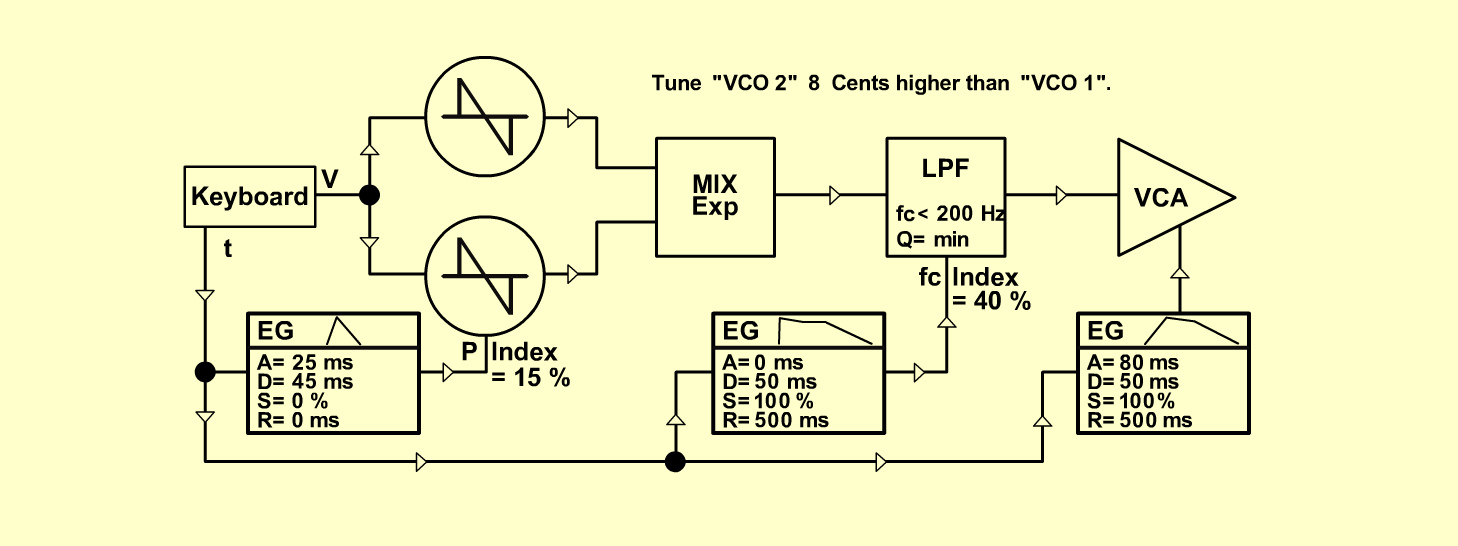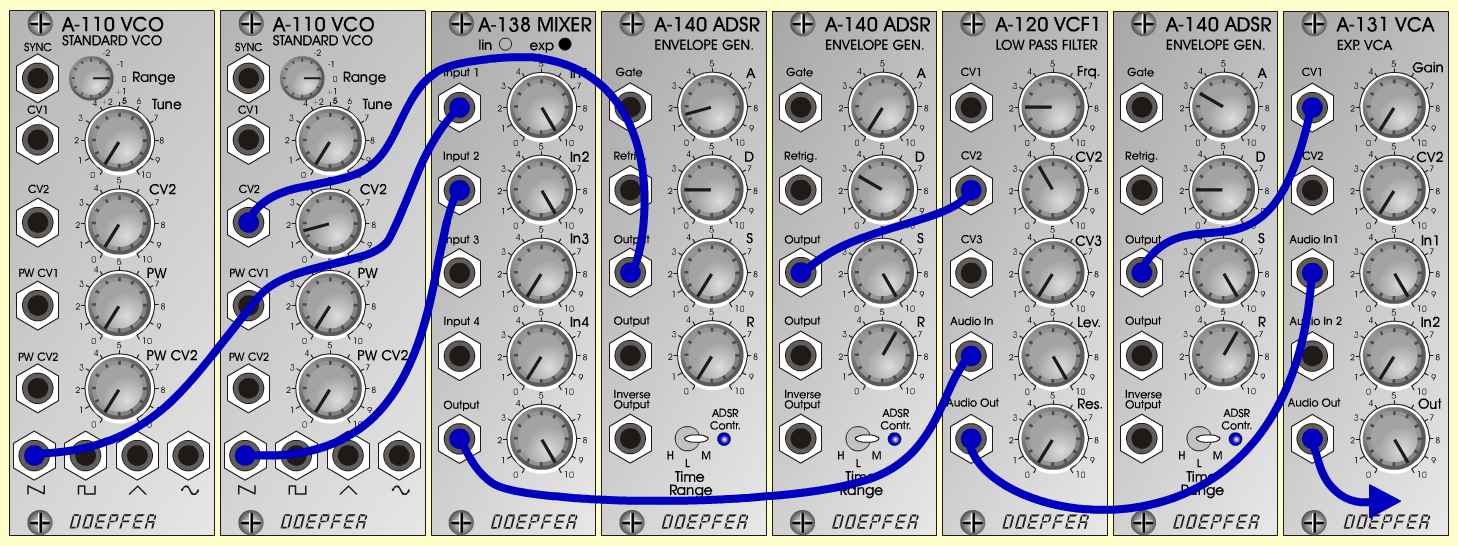Brass-PadInstrumentsD o e p f e r A - 100 Connections: Settings: A-110/1 (Saw)  <=>  A-138 (Input 1)   A-110/2 (Saw)  <=>  A-138 (Input 2)   A-138 (Output)  <=>  A-120 (Audio In)   A-120 (Audio Out)  <=>  A-131 (Audio In)   A-140/1 (Output)  <=>  A-110 (CV 2)   A-140/2 (Output)  <=>  A-120 (CV 2)   A-140/3 (Output)  <=>  A-131 (CV 1)   A-131 (Audio Out)  <=> Amplifier   Pitch, Trigger and Gate: KB A-110/2 (CV 2 = 1.5)   A-138 (In 1 = 10, In 2 = 10, Out = 10)   A-120 (Freq = 2, CV 2 = 4, Lev = 10, Res = 0)   A-131 (Gain = 0, Audio In 1 = 10,  Audio Out = 10)   A-140/1 (A = 1.5, D = 2, S = 0, R = 0, Range = M)   A-140/2 (A = 0, D = 3, S = 10, R = 6, Range = M)   A-140/3 (A = 3, D = 2, S = 10, R = 6, Range = M) Notes: Tune "VCO 2" 8  Cents higher than "VCO 1". Josef MuellerSound samples Brass-Pad chords Brass-Pad